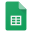# 15 Linear Functions

### Topic Description

During this work with linear patterns and contexts, students begin to surface ideas about the two parameters of a linear relationship: constant rate of change (slope) and initial value (y-intercept) and gain a conceptual understanding of the slope-intercept form of a linear equation.After exploring the rate of change of a linear relationship, students are introduced to the concept of slope and use the properties of dilations to show that the slope is the same between any two distinct points on a non-vertical line. Finally, students synthesize concepts learned and derive the equation of a line.

### Hook

Use spreadsheet software make it easy to determine the slope, the y-intercept, and the linear function for set of data points. An app will also be created that will the aid in the calculation of additional point of data. It can also be used to predict future or addition points of data.

### Teacher Documents

As part of the project, documents are provided to help implementing the analysis of data collected using a spreadsheet to plot the data and to generate the linear function using a Trendline on the graph of the data.

1. "Data, Scatter Chart, Trendlines, & Functions" document provides instructions for teaching and helping students collect data, graph it, analysis it, and create an app using Code.org's App Lab to build a generic Linear Function app or a specific app for a linear equation. Also included are additional problems that can be coded.
2. "Utah Population" a Google Sheet provides sample data that can be used to generate a linear equation to project future population growth in Utah.
3. "Delta Population" a Google Sheet provides sample data that can be used to generate a linear equation to project future population growth in Delta, Utah.
4. "Linear Function Calc" provides a sample layout for a Code.org App Lab app with a screenshoot and code.
5. Sample "Linear Function Calculator" app link.
6. Link to Utah Coding Project, "15 Coding Linear Functions" folder with all the related documents.
7. Link to recording of cricket chips and temperature for data to create a calculator to determine the temperature based on the number of cricket chirps for a given period of time. Link to recordings of Cricket Chirps.15 Coding Linear FunctionsUtah PopulationDelta PopulationLinear Function Calc